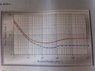# Reynolds Number and Power Number Problem

• cheerspens

## Homework Statement

If the Reynolds number is 4000, what is the Power (P) required in units of watts at a shaft speed (n) of 0.03 Hz? Assume the system contains acetone, with a kinematic viscosity of 0.419 stokes. The density of acetone is 0.785 grams per cubed centimeter. Us Curve B in the graph (attached as an image) to determine your answer. (Hint: Use the Reynolds number of the system to first calculate the diameter, then find the power number, and then calculate the power.

## Homework Equations

Re = D2n$\rho$ / $\mu$

Np = P / $\rho$n3D5

## The Attempt at a Solution

When trying to perform the calculation to determine the diameter, I could not get the grams to cancel out to get an answer in the proper units. While trying to continue to work through the problem I got an answer for the diameter to be 2.67 m. This doesn't seem right. How do I go about solving this problem?

#### Attachments

•photo.jpg
36.7 KB · Views: 1,000

First, find out what units are used in the formulas.
Second, check the info given in the problem to see if the units used match the units required by the formulas.

How do you get the grams from the density to cancel out in the Reynolds number equation?

What are the units of the quantities in the formula for Reynolds number?

You won't be able to solve this problem successfully without coming to grips with the units of the quantities in the equations.

Re=unitless
D=meters
n=Hz=s-1
$\rho$=kg/m3
$\mu$=m2/s

Where do the kilograms cancel out in this equation?
I was correct to convert cm to m and g to kg right?

You don't have the right units for mu. Mu has units of kg/m/s.